# Volume and Surface area of Solids

CONTENT LIST

## Volume and Surface area of Solid

### Solid

The object having definite shape and size are called solid.

A solid occupies a definite shape.

Things to remember

Total surface area: The total surface area of an object is sum of area of all of the surfaces of the object.

Lateral surface area: The lateral surface area of an object is equal to area of all of the sides of the object, except its base and top.

Curved surface area: The curved surface area of an object is equal to area of curved surface of the object.

### Cuboid

Solids like matchbox, chalkbox, a tile, a book, an almirah, a room, etc., are in the shape of a cuboid.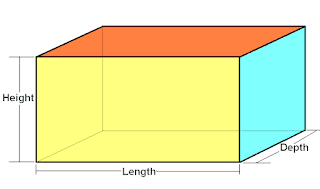Formulae for cuboid

length = l, breadth = b , height = h

i) Volume = l x b x h

ii) Total surface area = 2(lb + bh + lh)

iii) Lateral surface area = 2(l + b) x h

iv) Diagonal = √(l² + b² + h²)

### Cube

Solids like ice cube, sugar cube, dice, etc., are in the shape of a cube.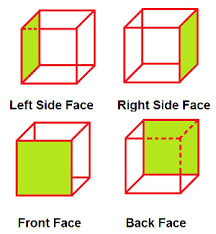Formulae for cube

length of each side = a

i) Volume = a³

ii) Total surface area = 6a²

iii) Lateral surface area = 4a²

iv) Diagonal = (√3)a

### Cylinder

Solids like jars, water bottle, pvc pipe, tumbler, etc., are in the shape of a cylinder.Formulae for cylinder

radius = r, height = h

i) Volume = πr²h

ii) Total surface area = 2πr(h + r)

iii) Curved surface area = 2πrh

### Hollow Cylinder

Solids like iron pipes, rubber tubes, pvc pipe, etc., are in the shape of a hollow cylinder.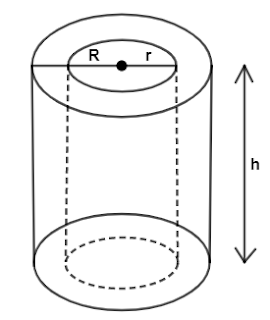Formulae for hollow cylinder

i) Volume = πh(R² - r²)

ii) Total surface area = 2π(R + r)(h + R - r)

iii) Curved surface area = 2πh(R + r)

### Cone

Solids like ice-cream cone, conical tent, funnels, etc., are in the shape of a cone.Formulae for cone

radius = r, height = h, slant height = l = √(h² + r²)

i) Volume = (1/3)πr²h

ii) Total surface area = πr(l + r)

iii) Curved surface area = πrl

### Sphere

Solids like football, cricket ball, etc., are in the shape of a sphere.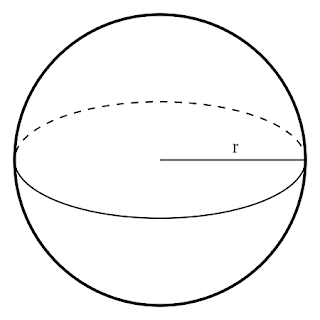Formulae for sphere

i) Volume = (4/3)πr³

ii) Surface area = 4πr²

### Hemisphere

Solids which are half of sphere is known as hemisphere.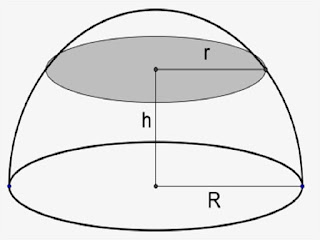Formulae for hemisphere

i) Volume = (2/3)πr³

ii) Curved surface area = 2πr²

ii) Total Surface area = 3πr²

### Spherical shellFormulae for spherical shell